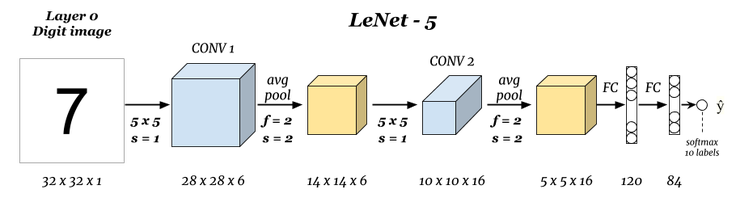## 深度学习：卷积神经网络构建

5374 字 · 175 阅读 · 2023 年 05 月 29 日### 知识点

• TensorFlow 高阶 API 构建
• TensorFlow 低阶 API 构建
• PyTorch 低阶 API 构建
• PyTorch 高阶 API 构建### 数据预处理

# 直接运行下载数据文件
wget -nc "http://labfile.oss.aliyuncs.com/courses/1081/MNIST_data.zip"
!unzip -o "MNIST_data.zip"


• 训练样本：train-images-idx3-ubyte.gz
• 训练标签：train-labels-idx1-ubyte.gz
• 测试样本：t10k-images-idx3-ubyte.gz
• 测试标签：t10k-labels-idx1-ubyte.gz

# 此段数据读取代码无需掌握
import gzip
import numpy as np

with gzip.open("MNIST_data/" + labels_path, 'rb') as labelsFile:

with gzip.open("MNIST_data/" + images_path, 'rb') as imagesFile:
X = np.frombuffer(imagesFile.read(), dtype=np.uint8, offset=16) \
.reshape(len(y), 784) \
.reshape(len(y), 28, 28, 1)

return X, y

train = {}
test = {}

'train-images-idx3-ubyte.gz', 'train-labels-idx1-ubyte.gz')
't10k-images-idx3-ubyte.gz', 't10k-labels-idx1-ubyte.gz')

train['X'].shape, train['y'].shape, test['X'].shape, test['y'].shape


from matplotlib import pyplot as plt
%matplotlib inline

plt.imshow(train['X'].reshape(28, 28), cmap=plt.cm.gray_r)# 样本 padding 填充
X_train = np.pad(train['X'], ((0, 0), (2, 2), (2, 2), (0, 0)), 'constant')
X_test = np.pad(test['X'], ((0, 0), (2, 2), (2, 2), (0, 0)), 'constant')
# 标签独热编码
y_train = np.eye(10)[train['y'].reshape(-1)]
y_test = np.eye(10)[test['y'].reshape(-1)]

X_train.shape, X_test.shape, y_train.shape, y_test.shape


from matplotlib import pyplot as plt
%matplotlib inline

plt.imshow(X_train.reshape(32, 32), cmap=plt.cm.gray_r)


### TensorFlow 高阶 tf.keras 构建

• tf.keras.layers.Conv1D ：一般用于文本或时间序列上的一维卷积。
• tf.keras.layers.Conv2D ：一般用于图像空间上的二维卷积。
• tf.keras.layers.Conv3D 。一般用于处理视频等包含多帧图像上的三维卷积。

• filters: 卷积核数量，整数。
• kernel_size: 卷积核尺寸，元组。
• strides: 卷积步长，元组。
• padding: "valid""same"import tensorflow as tf

model = tf.keras.Sequential()  # 构建顺序模型

# 卷积层，6 个 5x5 卷积核，步长为 1，relu 激活，第一层需指定 input_shape
activation='relu', input_shape=(32, 32, 1)))
# 平均池化，池化窗口默认为 2
# 卷积层，16 个 5x5 卷积核，步为 1，relu 激活
5, 5), strides=(1, 1), activation='relu'))
# 平均池化，池化窗口默认为 2
# 需展平后才能与全连接层相连
# 全连接层，输出为 120，relu 激活
# 全连接层，输出为 84，relu 激活
# 全连接层，输出为 10，Softmax 激活
# 查看网络结构
model.summary()


# 编译模型，Adam 优化器，多分类交叉熵损失函数，准确度评估

# 模型训练及评估
model.fit(X_train, y_train, batch_size=64, epochs=2, validation_data=(X_test, y_test))


### TensorFlow 低阶 tf.nn 构建

TensorFlow 低阶 API 主要会用到 tf.nn 模块，通过构建前向计算图后，再建立会话完成模型训练。其中，卷积函数为 tf.nn.conv2d ，平均池化函数为 tf.nn.avg_pool 。值得注意的是，虽然大部分参数含义上和 Keras 相似，但是用法上却不同，大家需要根据下面的示例代码并结合官方文档学习。

class Model(object):
def __init__(self):
# 随机初始化张量参数
self.conv_W1 = tf.Variable(tf.random.normal(shape=(5, 5, 1, 6)))
self.conv_W2 = tf.Variable(tf.random.normal(shape=(5, 5, 6, 16)))
self.fc_W1 = tf.Variable(tf.random.normal(shape=(5*5*16, 120)))
self.fc_W2 = tf.Variable(tf.random.normal(shape=(120, 84)))
self.out_W = tf.Variable(tf.random.normal(shape=(84, 10)))

self.fc_b1 = tf.Variable(tf.zeros(120))
self.fc_b2 = tf.Variable(tf.zeros(84))
self.out_b = tf.Variable(tf.zeros(10))

def __call__(self, x):
x = tf.cast(x, tf.float32)  # 转换输入数据类型
# 卷积层 1: Input = 32x32x1. Output = 28x28x6.
conv1 = tf.nn.conv2d(x, self.conv_W1, strides=[1, 1, 1, 1], padding='VALID')
# RELU 激活
conv1 = tf.nn.relu(conv1)
# 池化层 1: Input = 28x28x6. Output = 14x14x6.
pool1 = tf.nn.avg_pool(conv1, ksize=[1, 2, 2, 1], strides=[1, 2, 2, 1], padding='VALID')
# 卷积层 2: Input = 14x14x6. Output = 10x10x16.
conv2 = tf.nn.conv2d(pool1, self.conv_W2, strides=[1, 1, 1, 1], padding='VALID')
# RELU 激活
conv2 = tf.nn.relu(conv2)
# 池化层 2: Input = 10x10x16. Output = 5x5x16.
pool2 = tf.nn.avg_pool(conv2, ksize=[1, 2, 2, 1], strides=[1, 2, 2, 1], padding='VALID')
# 展平. Input = 5x5x16. Output = 400.
flatten = tf.reshape(pool2, [-1, 5*5*16])
# 全连接层
return outs


def loss_fn(model, x, y):
preds = model(x)
return tf.reduce_mean(tf.nn.softmax_cross_entropy_with_logits(logits=preds, labels=y))

def accuracy_fn(logits, labels):
preds = tf.argmax(logits, axis=1)  # 取值最大的索引，正好对应字符标签
labels = tf.argmax(labels, axis=1)
return tf.reduce_mean(tf.cast(tf.equal(preds, labels), tf.float32))


from sklearn.model_selection import KFold
from tqdm.notebook import tqdm

EPOCHS = 2  # 迭代此时
BATCH_SIZE = 64  # 每次迭代的批量大小
LEARNING_RATE = 0.001  # 学习率

model = Model()  # 实例化模型类

for epoch in range(EPOCHS):  # 设定全数据集迭代次数
indices = np.arange(len(X_train))  # 生成训练数据长度规则序列
np.random.shuffle(indices)  # 对索引序列进行打乱，保证为随机数据划分
batch_num = int(len(X_train)/BATCH_SIZE)  # 根据批量大小求得要划分的 batch 数量
kf = KFold(n_splits=batch_num)  # 将数据分割成 batch 数量份
# KFold 划分打乱后的索引序列，然后依据序列序列从数据中抽取 batch 样本
for _, index in tqdm(kf.split(indices), desc='Training'):
X_batch = X_train[indices[index]]  # 按打乱后的序列取出数据
y_batch = y_train[indices[index]]

with tf.GradientTape() as tape:  # 追踪梯度
loss = loss_fn(model, X_batch, y_batch)

trainable_variables = [model.conv_W1, model.conv_W2,
model.fc_W1, model.fc_W2, model.out_W,
model.fc_b1, model.fc_b2, model.out_b]  # 需优化参数列表

# 每一次 Epoch 执行小批量测试，防止内存不足
indices_test = np.arange(len(X_test))
batch_num_test = int(len(X_test)/BATCH_SIZE)
kf_test = KFold(n_splits=batch_num_test)
test_acc = 0
for _, index in tqdm(kf_test.split(indices_test), desc='Testing'):
X_test_batch = X_test[indices_test[index]]
y_test_batch = y_test[indices_test[index]]
batch_acc = accuracy_fn(model(X_test_batch), y_test_batch)  # 计算准确度
test_acc += batch_acc  # batch 准确度求和

accuracy = test_acc / batch_num_test  # 测试集准确度
print(f'Epoch [{epoch+1}/{EPOCHS}], Accuracy: [{accuracy:.2f}], Loss: [{loss:.4f}]')


### PyTorch 低阶 nn.Module 构建

import torch
import torch.nn as nn
import torch.nn.functional as F

class LeNet(nn.Module):
def __init__(self):
super(LeNet, self).__init__()
# 卷积层 1
self.conv1 = nn.Conv2d(
in_channels=1, out_channels=6, kernel_size=(5, 5), stride=1)
# 池化层 1
self.pool1 = nn.AvgPool2d(kernel_size=(2, 2))
# 卷积层 2
self.conv2 = nn.Conv2d(
in_channels=6, out_channels=16, kernel_size=(5, 5), stride=1)
# 池化层 2
self.pool2 = nn.AvgPool2d(kernel_size=(2, 2))
# 全连接层
self.fc1 = nn.Linear(in_features=5*5*16, out_features=120)
self.fc2 = nn.Linear(in_features=120, out_features=84)
self.fc3 = nn.Linear(in_features=84, out_features=10)

def forward(self, x):
x = F.relu(self.conv1(x))
x = self.pool1(x)
x = F.relu(self.conv2(x))
x = self.pool2(x)
x = x.reshape(-1, 5*5*16)
x = F.relu(self.fc1(x))
x = F.relu(self.fc2(x))
x = F.softmax(self.fc3(x), dim=1)
return x


model = LeNet()
model


model(torch.Tensor(X_train).reshape(-1, 1, 32, 32))


import torch.utils.data

# 依次传入样本和标签张量，制作训练数据集和测试数据集
train_data = torch.utils.data.TensorDataset(torch.Tensor(
X_train), torch.Tensor(train['y']))
test_data = torch.utils.data.TensorDataset(torch.Tensor(
X_test), torch.Tensor(test['y']))

train_data, test_data


train_loader = torch.utils.data.DataLoader(train_data, batch_size=64, shuffle=True)



loss_fn = nn.CrossEntropyLoss()  # 交叉熵损失函数


def fit(epochs, model, opt):
# 全数据集迭代 epochs 次
print('================ Start Training =================')
for epoch in range(epochs):
# 从数据加载器中读取 Batch 数据开始训练
for i, (images, labels) in enumerate(train_loader):

images = images.reshape(-1, 1, 32, 32)  # 对特征数据展平，变成 784
labels = labels.type(torch.LongTensor)  # 真实标签

outputs = model(images)  # 前向传播
loss = loss_fn(outputs, labels)  # 传入模型输出和真实标签

loss.backward()  # 从最后 loss 开始反向传播
opt.step()  # 优化器迭代

# 自定义训练输出样式
if (i+1) % 100 == 0:
print('Epoch [{}/{}], Batch [{}/{}], Train loss: [{:.3f}]'

# 每个 Epoch 执行一次测试
correct = 0
total = 0
images = images.reshape(-1, 1, 32, 32)
labels = labels.type(torch.LongTensor)

outputs = model(images)
# 得到输出最大值 _ 及其索引 predicted
_, predicted = torch.max(outputs.data, 1)

correct += (predicted == labels).sum().item()  # 如果预测结果和真实值相等则计数 +1
total += labels.size(0)  # 总测试样本数据计数

print('============= Test accuracy: {:.3f} =============='.format(
correct / total))


fit(epochs=2, model=model, opt=opt)


### PyTorch 高阶 nn.Sequential 构建

class Flatten(nn.Module):
def forward(self, input):
return input.reshape(input.size(0), -1)

# 构建 Sequential 容器结构
model_s = nn.Sequential(
nn.Conv2d(1, 6, (5, 5), 1),
nn.ReLU(),
nn.AvgPool2d((2, 2)),
nn.Conv2d(6, 16, (5, 5), 1),
nn.ReLU(),
nn.AvgPool2d((2, 2)),
Flatten(),
nn.Linear(5*5*16, 120),
nn.ReLU(),
nn.Linear(120, 84),
nn.ReLU(),
nn.Linear(84, 10),
nn.Softmax(dim=1)
)

model_s


opt_s = torch.optim.Adam(model_s.parameters(), lr=0.001)  # Adam 优化器
fit(epochs=2, model=model_s, opt=opt_s)


### 系列文章•